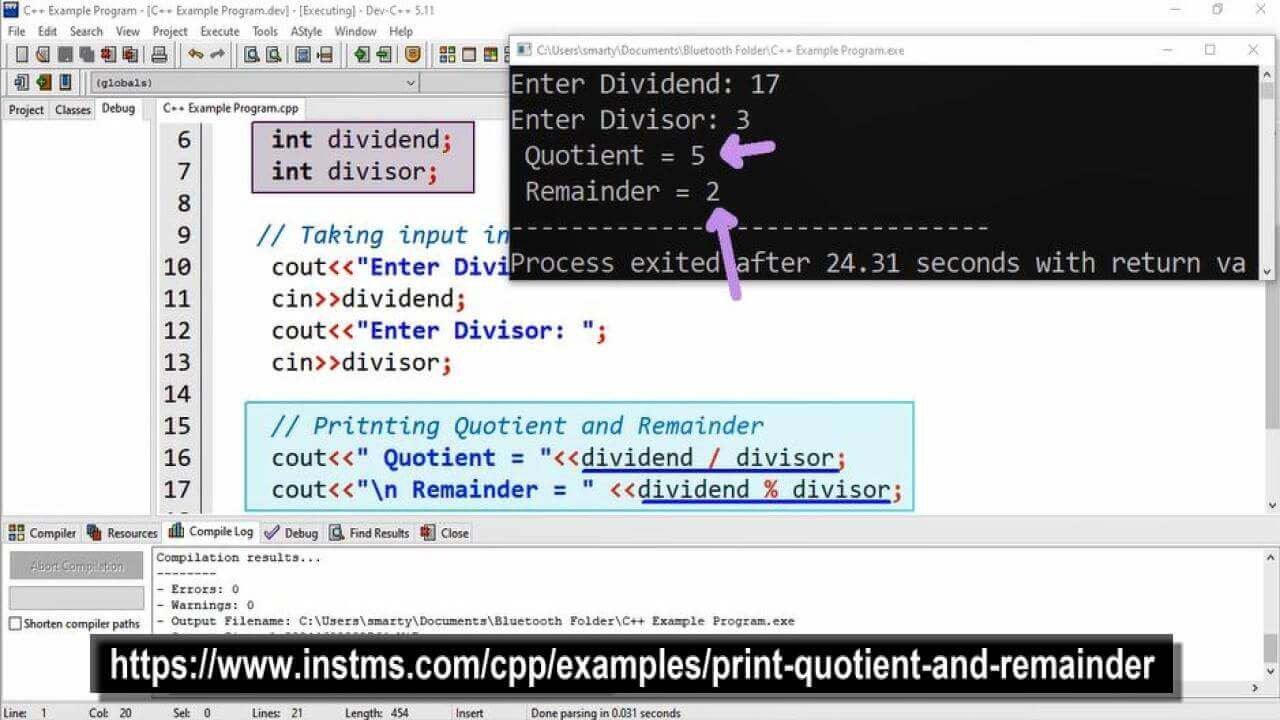# Print Quotient And Remainder Program Example using CPP

## Source Code

#include <iostream>

using namespace std;

int main(int argc, char** argv) {

// Variable Declaration for input
int dividend;
int divisor;

// Taking input in Variables
cout<<"Enter Dividend: ";
cin>>dividend;
cout<<"Enter Divisor: ";
cin>>divisor;

// Pritnting Quotient and Remainder
cout<<" Quotient = "<<dividend / divisor;
cout<<"\n Remainder = " <<dividend % divisor;

return 0;
}

## Output## Working

In this program two variables of int type are used, named as dividend and divisor. Dividend will be divided by divisor to get quotient. Again this dividend will get modulus by divisor to get remainder.

After declaration we will take input in these variables using cin statement and then get print quotien,remainder using cout statement.